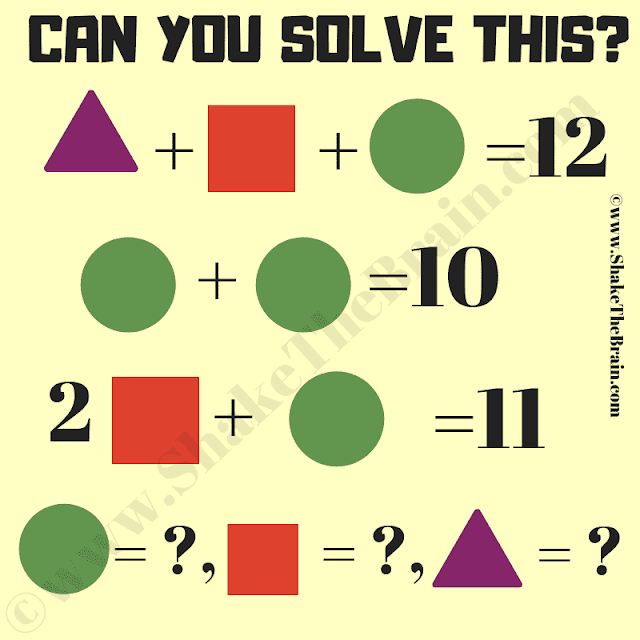Challenge your mind with a mathematical brain teaser: an algebra problem. Engage in this captivating puzzle and find the solution.

How good are you at Mathematics? Let us test your Maths skills by solving these simple Maths equations. In this math equation brain teaser, you are shown some algebraic equations where variables are represented by different geometrical shapes. Can you find the values of Triangle, Square, and Circle in the following Maths equations?Can you solve this math equations brain teaser?